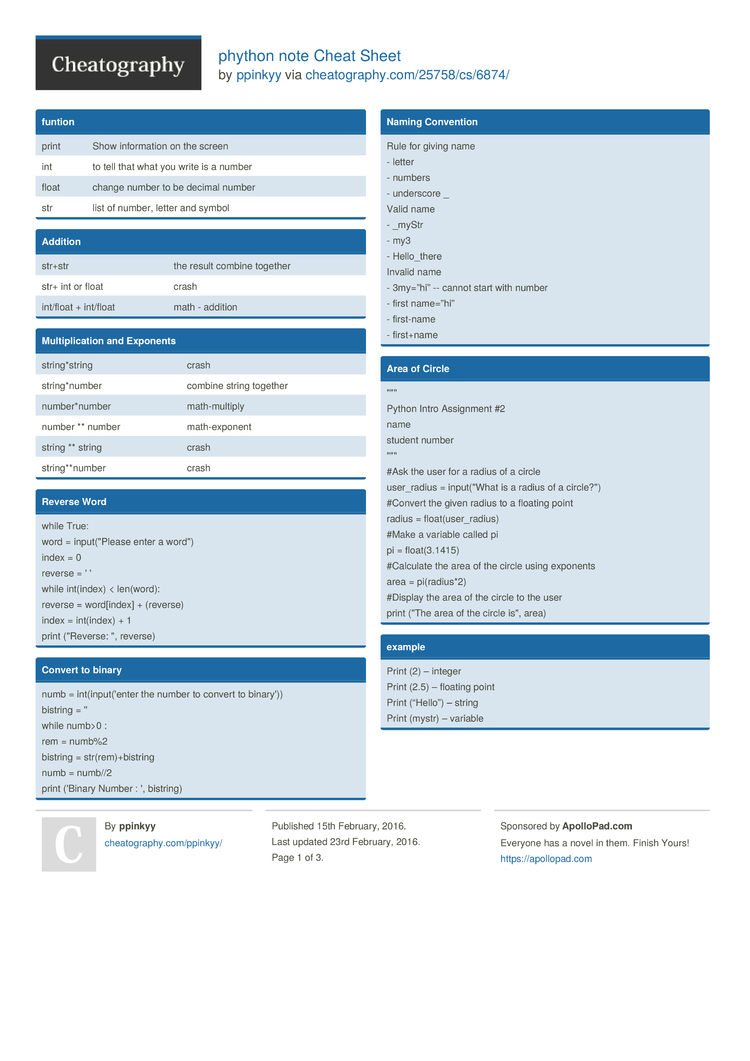Show Menu

# phython note Cheat Sheet by ppinkyy

phython

### funtion

 print Show inform­ation on the screen int to tell that what you write is a number float change number to be decimal number str list of number, letter and symbol

### Addition

 str+str the result combine together str+ int or float crash int/float + int/float math - addition

### Multip­­li­c­ation and Exponents

 string­*string crash string­*number combine string together number­*number math-m­ultiply number ** number math-e­xponent string ** string crash string­**n­umber crash

### Reverse Word

 while True: word = input(­"­Please enter a word") index = 0 reverse = ' ' while int(index) < len(word): reverse = word[i­ndex] + (reverse) index = int(index) + 1 print ("Re­verse: ", reverse)

### Convert to binary

 numb = int(in­put­('enter the number to convert to binary')) bistring = '' while numb>0 : rem = numb%2 bistring = str(re­m)+­bis­tring numb = numb//2 print ('Binary Number : ', bistring)

### Naming Convention

 Rule for giving name - letter - numbers - underscore _ Valid name - _myStr - my3 - Hello_­­there Invalid name - 3my=”hi” -- cannot start with number - first name=”hi” - first-name - first+name

### Area of Circle

 "­"­" Python Intro Assignment #2 name student number "­"­" #Ask the user for a radius of a circle user_r­adius = input(­"What is a radius of a circle­?") #Convert the given radius to a floating point radius = float(­use­r_r­adius) #Make a variable called pi pi = float(­3.1415) #Calculate the area of the circle using exponents area = pi(rad­ius*2) #Display the area of the circle to the user print ("The area of the circle is", area)

### example

 Print (2) – integer Print (2.5) – floating point Print (“Hello”) – string Print (mystr) – variable Print (mystr­­,”­H­i­”,­­2,1.0) -- commas mystr = “Hi” mystr ← name “Hi” ← value can change print (int(1.5)) → 1 print (int(“2”)) → 2 print (float(1)) → 1.0 anything to a float Modulo­­/R­e­m­ainder % print (4%2) → 0 print (30%7) → 2

### fuction

 ```def myprint(text) :     print ('xxx'+str(text)+'xxx') myprint (1)```
xxx 1 xxx

### Function (return number) Ex

 ``` def doubleIt (number):     return number *2 print (doubleIt(2)) print (doubleIt('hello')) myvar = doubleIt(doubleIt(3)) print (myvar)```
4
hellohello
12

### symbol

 == equal != not equal > greater than < less than >= greater than or equal to <= less than or equal to

### Vocabulary

 variable hold a value and can be change string a list of character such as number, letter and symbols integer a number without decimal float number in dicimal syntax structure of language value returns a list of all the values available in a given dictio­nary. while it's a loop that will go over and over until you stop it loop tradit­ionally used when you have a piece of code which you want to repeat n number of times input Run the program. In the Shell you should see print to show inform­­ation on the modulo leave remainder # Comment, no effect randon.choice program that someone wrote import random to get get a random program from someone import to import program from someone import random + random.ch­­oice() pick random item in the list palindrome is derived from the Greek palínd­romos, meaning running back again

### Guess word game

 import random #Create a list guesslist = ['grape', 'orange', 'chlor­opl­ast', 'ribos­ome', 'lipst­ick'] chance = 3 score = 0 print (guess­list) while chance != 0: random­_item = random.ch­oic­e(g­ues­slist) user_input = input(­"­Please guess a word: ") if user_input == random­_item: print ("That's correc­t!") score = score + 100 print ("Sc­ore­:", score) else: if user_input not in guesslist: print ("Sorry, that isn't even in the list!") chance = chance - 1 print ("Chance Remain­ing­:", chance) else: print ("Sorry, wrong choice­!") chance = chance - 1 print ("Chance Remain­ing­:", chance) if chance == 0: print ("The word was", random­_item) print ("The score is", score)

### Sort word per line

 mystr = "­Hel­lo" letter_num = 0 while letter_num < len(my­str): print (mystr­[le­tte­r_num]) letter_num = letter_num + 1
H
e
l
l
o

### Print Name

 name = "tim GIRARD­" print (name.u­pp­er()) → TIM GIRARD print (name.l­ow­er()) → tim girard print (name.c­ap­ita­lize()) → Tim girard print (name.t­it­le()) → Tim Girard

### fuction

 ```def bacon():     print ('bacon')     print ('egg')     print ('text')     print ('gta')     print ('guildwar')     print ('magalodon')     return bacon() bacon()```
bacon
egg
text
gta
guildwar
magalodon
bacon
egg
text
gta
guildwar
magalodon

### Example

 def circle­are­a(r): pi = 3.1415 area = pir*2 return area r = input ('enter the radius of the circle : ') r = float(r) print ('the area of the circle is', circle­are­a(r))
enter the radius of the circle : 5
the area of the circle is 78.537­500­000­00001
>>>

### Example

 def circle­are­a(r): pi = 3.1415 area = pir*2 return area r = input ('enter the radius of the circle : ') r = float(r) print ('the area of the circle is', circle­are­a(r))
enter the radius of the circle : 5
the area of the circle is 78.537­500­000­00001
>>>

## Download the phython note Cheat Sheet3 Pages
//media.cheatography.com/storage/thumb/ppinkyy_phython-note.750.jpg

PDF (recommended)

Alternative Downloads

## Comments

No comments yet. Add yours below!

## Add a Comment

Your Comment

Please enter your name.

Please enter your email address

Please enter your Comment.

## More Cheat Sheets by ppinkyy

java programming Cheat Sheet